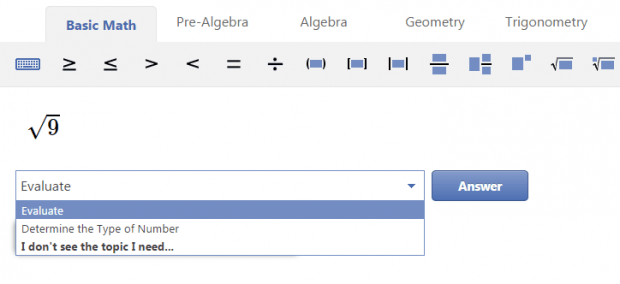# How to solve math problems online [Tip]

Whether you’re a student or an established professional, you definitely cannot evade Math. It’s part of your everyday life. In the workplace, there are certain tasks that would require you to solve basic and advanced mathematical equations. At school, you need to do well in Algebra, Geometry, Trigonometry, Calculus, etc.

If you find it very easy and exciting to solve various Math problems, then you’re quite gifted. However, there are also those who are struggling to remember even the simplest Mathematical formulas and are too reliant on their scientific calculators. If you belong to the latter, then this post is for you.

### How to solve math problems online

Did you know that there’s a web app that lets you solve basic and advanced mathematical problems online? So if you want to make use of it, here’s what you need to do:

• Next, go here to open the homepage of “Mathway” – a web app for solving various kinds of mathematical equations.
• Once you’re on Mathway, you’ll see an interface wherein you can enter and solve your mathematical problems.
• In the top navigation, you’ll see different tabs. Each tab represents a particular mathematical field or category including Basic Math, Pre-Algebra, Algebra, Geometry, Calculus, etc. So just choose your preferred category then proceed to the next step.
• Based on your chosen category, the toolbar below it will show relevant tools and options. These are the tools that you can use to formulate your mathematical problem. For example, if you choose Basic Math, the toolbar will show a fraction tool, square root tool, exponent tool, etc.• Moving on, you just need to use the tools provided to formulate your mathematical problem in the editor.• Thereafter, a drop-down menu will appear right below your mathematical problem. So just choose your desired action (ex. evaluate, solve for x) then click “Answer”.
• Wait while the app solves your problem and in just a few seconds, it will show you the result. If you log in, you can even view the step-by-step solution as to how the web app managed to come up with such answer.

It’s that simple.

Just keep in mind that Mathway is primarily intended as a tool to aid you solve various Math problems. However, you shouldn’t be too dependent on it. You shouldn’t also use it as a way to cheat on your tests.